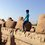# 0-1 String Problem

I recently had a problem in my mind and am having some trouble proving my solution, have a look.

Imagine I make a string of numbers with only 1's and 0's. ex: $10101010000111010101...$

$Q:$ How many numbers (1's and 0's) would I have to write (at least) to guarantee a repetition of any $n$-string number. Ex: Let $n=2$, generate a random sequence of 1's and 0's: $100110$. Notice that the first 2 digits are "$10$", so is the 5th and 6th "$10$" a repetition!

For $n=2$, I have proved a string of length $>5$ must have at least one repetition. For $n=2$ we have answer $5$. Similarly, for $n=3$, we found the answer to be $10$, the string length cannot exceed $10$ without repeating a $3$-string number. I couldn't find a number for $n=4$ but I have shown that for any $n$ the string length does not exceed $2^n+n-1$ but I suspect $2^n+n-1$ might be the general formula (If you substitute $n=2$ and $n=3$ you will find the results match), but I haven't been able to prove this for all $n$.

PS: I think the solution might be related to graph theory.Note by Apratim Ghosh
3 years, 4 months ago

This discussion board is a place to discuss our Daily Challenges and the math and science related to those challenges. Explanations are more than just a solution — they should explain the steps and thinking strategies that you used to obtain the solution. Comments should further the discussion of math and science.

When posting on Brilliant:

• Use the emojis to react to an explanation, whether you're congratulating a job well done , or just really confused .
• Ask specific questions about the challenge or the steps in somebody's explanation. Well-posed questions can add a lot to the discussion, but posting "I don't understand!" doesn't help anyone.
• Try to contribute something new to the discussion, whether it is an extension, generalization or other idea related to the challenge.

MarkdownAppears as
*italics* or _italics_ italics
**bold** or __bold__ bold
- bulleted- list
• bulleted
• list
1. numbered2. list
1. numbered
2. list
Note: you must add a full line of space before and after lists for them to show up correctly
paragraph 1paragraph 2

paragraph 1

paragraph 2

[example link](https://brilliant.org)example link
> This is a quote
This is a quote
    # I indented these lines
# 4 spaces, and now they show
# up as a code block.

print "hello world"
# I indented these lines
# 4 spaces, and now they show
# up as a code block.

print "hello world"
MathAppears as
Remember to wrap math in $$ ... $$ or $ ... $ to ensure proper formatting.
2 \times 3 $2 \times 3$
2^{34} $2^{34}$
a_{i-1} $a_{i-1}$
\frac{2}{3} $\frac{2}{3}$
\sqrt{2} $\sqrt{2}$
\sum_{i=1}^3 $\sum_{i=1}^3$
\sin \theta $\sin \theta$
\boxed{123} $\boxed{123}$

Sort by:

For n=2, surely this is 6?

- 3 years, 4 months ago

This is a well-studied problem. Maybe try OEIS first next time: http://oeis.org/A052944

- 3 years, 4 months ago